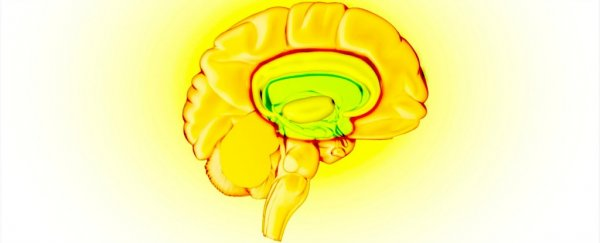# Do Great Mathematicians Have Different Brains to The Rest of Us?

JOSH HRALA
6 MAY 2016

How did great mathematicians like Stephen Hawking and Albert Einstein make the leap from understanding basic mathematical concepts to answering fundamental questions about the Universe? Is this level of thinking the product of hard work, or are the brains of great thinkers inherently different than the rest of ours?

If these sound like questions you’ve had in the past, fear not, because neuroscientists have been pondering them for years.

For researchers, the debate has largely been about which areas of the brain are at work when mathematicians think about particularly complex issues - specifically, if they use the same areas to process both math and language.

Now, a new study has put the debate to rest once and for all by identifying a special area in the brain that's used for mathematical thinking - and even non-maths experts are born with it.

In the study, conducted by researchers from the University of Paris, France, 15 high-level mathematicians and 15 high-level academics in other fields underwent fMRI scans and were asked to answer 'true' or 'false' to a series of questions. Some of these questions were mathematical, and some were word-based.

The team found that word-based problems triggered pathways typically associated with language processing, but when the questions prompted the participants to use high-level mathematical processing, the parietal, the prefrontal, and inferior temporal regions in the brains of the mathematicians lit up, according to Bob Yirka at Medical Xpress.

The brains of non-maths academics didn't light up, most likely because they weren't able to understand the problems being presented.

"[B]ecause they were not trained in higher level math, the same areas in the brains of the non-mathematicians lit up only when asked more general questions about numbers and math formulas," says Yirka

So what does this all mean, especially for us non-geniuses? Well, it shows that maths is processed in a specific area of the brain, no matter how complex the questioning.

For example, when Stephen Hawking is balancing his cheque book, he's using the same parts of his brain that he would while calculating what happens at a black hole's event horizon, which is pretty neat, because those are two completely different scenarios with completely different levels of mathematical thinking.

The real question now is whether non-mathematicians can learn to understand high-level math through training, and, if they can, if the same areas start to light up as their comprehension increases.

Daniel Ansari, a cognitive neuroscientist from the University of Western Ontario in Canada, who was not a part of the study, summed up this question to Scientific American’s Jordana Cepelewicz

"Most of us master basic arithmetic, so we’re already recruiting these brain regions, but only a fraction of us go on to do high-level math. We don’t yet know whether becoming a mathematical expert changes the way you do arithmetic or whether learning arithmetic lays out the foundation for acquiring higher-level mathematical concepts."

So far, there's no word if such a study will be carried out, but it seems like the logical next step for researchers trying to understand the minds of some of our time’s greatest theorists.

The team’s findings were published in Proceedings of the National Academy of Sciences.